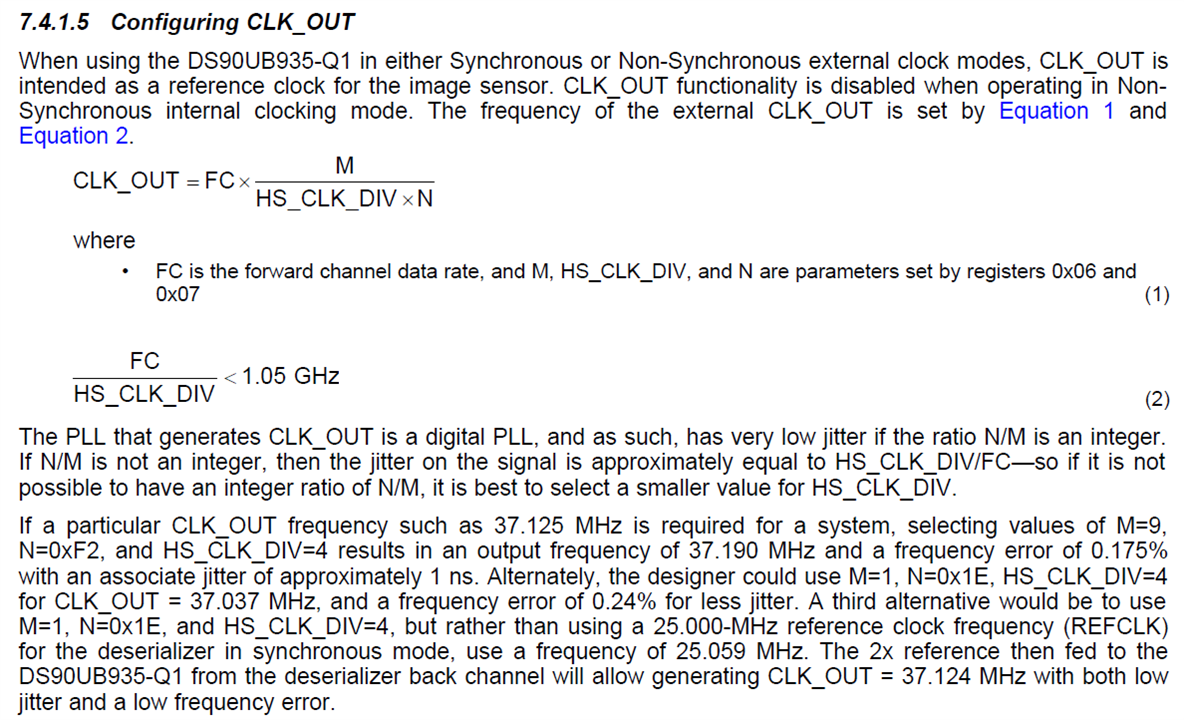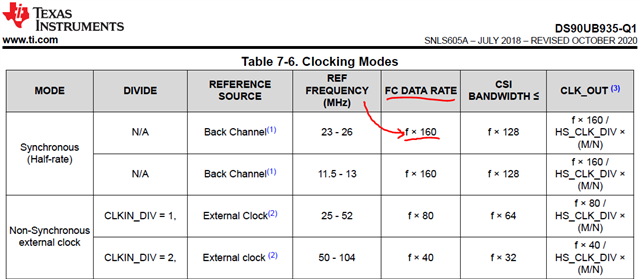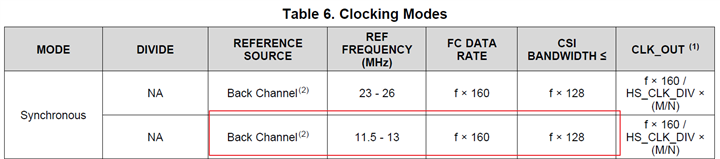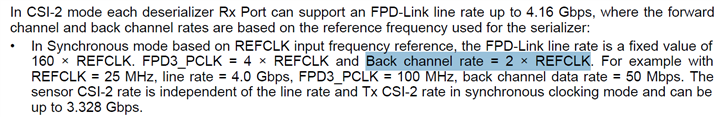If you have a related question, please click the "Ask a related question" button in the top right corner. The newly created question will be automatically linked to this question.

# DS90UB935-Q1: CLKOUT computation

Part Number: DS90UB935-Q1

Dear team,

My customer wants to use DS90UB935-Q1's CLKOUT as a reference clock to the image sensor. And the reference clock they need is 24MHz. Our 935's default value is 25MHz. We have an example as below, but I am a little confused for the computation result. In below example, CLKOUT=37.125MHz, selecting values of M=9, N=0xF2(362), and HS_CLK_DIV=4 results in an output frequency of 37.190 MHz. CLKOUT=FC*M/(HS_CLK_DIV*N)=FC*9/(4*362)=0.00622*FC. FC should be 4Gbps in sync mode, so CLKOUT=0.00622*FC=0.00622*4Gbps=0.00622*2GHz=12.44MHz. The result is 12.44MHz instead of 37.19MHz. Could you please tell me which step is wrong? I need to help customer to compute 24MHz CLKOUT.Thanks & Best Regards,

Sherry

• Hello Sherry,

In the CLK_OUT section for 935 datasheet there is an example for generating 37.037Mhz with HS_CLK_DIV = 4, M = 0x01, and N = 0x1E. The N value is a typo and should be 0x1B.

I noticed that you are not using the decimal value for "N=0xF2." (N=0xF2 (hex) =242 (dec); do not use 362 (oct)). Please use the decimal values instead of hex in your calculations.

Also, Please substitute 25 MHz for "f" to calculate the FC data rate. Then use "FC" in the CLK_OUT equation.Note that "The PLL that generates CLK_OUT is a digital PLL, and as such, has very low jitter if the ratio N/M is an integer.
If N/M is not an integer, then the jitter on the signal is approximately equal to HS_CLK_DIV/FC—so if it is not
possible to have an integer ratio of N/M, it is best to select a smaller value for HS_CLK_DIV."

In the case where your calculations of the N/M ratio do not lead to an integer value, you can vary the Ref Frequency (which will lead to a slightly different FC data rate) so that you can obtain an integer value for your N/M ratio, This will compromise the accuracy of the FC data rate, but provide you with a CLK_OUT with very low jitter. On the other hand, if your jitter tolerance is more lenient, and you can get away with some amount of jitter, your N/M ratio can be a non-integer value and you can maintain the accuracy of your FC data rate. These may be the trade-offs.

Hope this helps.

Best Regards,

Shruti More

• Hi Shruti,

Thanks for your reply! Very clear! But I still have two other questions,

1. In my understanding, f should be back channel rate. In the first line, back channel is 50Mbps, so f=25MHz. In the second line, back channel is 25Mbps, so f=12.5MHz. Therefore synchronous mode include two kinds of FC data rate, if BC data rate is 50Mbps, then FC=4Gbps; if BC data rate is 25Mbps, then FC=2Gbps. But in the 954 datasheet, BC data rate must be 2*REFCLK in the synchronous mode, so BC data rate only have one choice 50Mbps. It is contradictory. If BC data rate must be 50Mbps, then how do we get the second line data in the table 6?

2. Can we use the FC=2Gbps when we use CLKOUT as a reference clock to the image sensor in the sync mode?Thanks & Best Regards,

Sherry

• Hi Sherry,

When you select BC frequency to 25 Mbps, your BC data rate will be 1*REFCLK in the synchronous mode. There needs to be an update in the datasheet.

Yes, you can use the FC=2Gbps when you use CLKOUT as a reference clock to the image sensor in the sync mode.

Hope this helps.

Best Regards,

Shruti More